#### Chapter 22 Tabular Representation of Statistical Data R.D. Sharma Solutions for Class 9th Exercise 22.1

Exercise 22.1

1. What do you understand by the word ''statistics'' in
(i) Singular form ?
(ii) Plural form ?

Solution

(i) In singular form statistics may be defined as the science of collection, presentation, analysis and interpretation of numerical data.
(ii) In plural form statistics means numerical facts or observations collected with definite purpose.
For examples, the income and expenditure of persons in a particular locality, number of males and females in a particular town are statistics.

2. Describe some fundamental characteristic of statistics.

Solution

The plural form statistics has the simplest structure and the singular form statistics has many components. There is only structural difference between singular and plural form statistics. Some of the characteristics of a statistics are .
1. Statistics is a collection of observations. So, clearly a single observation cannot form a statistics.
2. Statistics are collected with definite purpose.
3. Statistics are comparable and classified into various types depending on their properties.
4. Statistics are expressed quantitatively and not qualitatively.

3. What are (i) primary data ? (ii) secondary data ? Which of the two - the primary or the secondary data − is more reliable and why ?

Solution

(i) When an investigator collects data himself with a definite plan or designs in his (her) mind, it is called primary data.
(ii) Data which are not originally collected rather obtained from published or unpublished sources are known as secondary data.

4. Why do we group data ?

Solution

To study the features of a collected data, the data must be arranged in a condensed form. There are a number of ways to arrange the data in condensed form, namely,
1. Serial order or alphabetical order
2. Ascending order
3. Descending order
But, if the number of observations is large, then arranging data in ascending or descending or serial order is a tedious job and it does not tell us much except perhaps the minimum(s) and maximum(s) of data. So to make it easily understandable and clear we condense the data into groups or table form.

5. Explain the meaning of the following terms:
(i) Variate
(ii) Class-integral
(iii) Class-size
(iv) Class-mark
(v) Frequency
(vi) Class limits
(vii) True class limits.

Solution

(i) Variate - Any character that can vary from one individual to another is called variate.
(ii) Class interval - In the data each group into which raw data is considered is called a class-interval.
(iii) Class-size - The difference between the true upper limit and lower limit is called the class size of that class size.
(iv) Class-mark -The middle value of the class is called as the class mark.Class mark = upper limit + lower limit / 2
(v) Frequency - The number of observations corresponding to class is called its frequency.
(vi) Class limits - Each class is bounded by two figures ,called the class limits .The figures on the left side of the classes are called lower limits while figures on the right side are called upper limits.
(vii) True class limits  - If classes are inclusive.Eg:15-19,20-24,25-29….
Then, true lower limit of class = upper limit of class-0.5
Eg:- True limits of the class is 15-19 are 14.5 and 19.5
But if classes are exclusive like 10-20,20-30,30-40…
Here class limits and true class limits are the same.

6. The ages of ten students of a group are given below. The ages have been recorded in years and months:
8 - 6, 9 - 0, 8 - 0,4, 9 - 3, 7 - 8, 8 - 11, 8 - 7, 9 - 2, 7 - 10, 8 - 8
(i) What is the lowest age?
(ii) What is the highest age?
(iii) Determine the range?

Solution

The ages of ten students of a group are given below
8-6,9-0,8-4,9-3,7-8,8-11,8-7,9-2,7-10,8-8.
(i) Lowest age is 7 years 8months
(ii) Highest age is  9 years,3 months
(iii) Range = Highest age-lowest age = 9 year,3 months,7 years,8 months = 1 year 7 months

7. The monthly pocket money of six friends is given below:
Rs 45, Rs 30, Rs 50, Rs 25, Rs 45
(i) What is the highest pocket money?
(ii) What is the lowest pocket money?
(iii) What is the range?
(iv) Arrange the amounts of pocket money in ascending order.

Solution

The monthly pocket money of six friends is given below:
Rs 45,Rs 30, Rs 40, Rs 25, Rs 45.
(i) Highest pocket money = Rs 50
(ii) Lowest pocket money = Rs 25
(iii) Range = 50-25 = 25
(iv) The amounts of pocket money in an ascending order is: Rs 25,Rs 30, Rs 40, Rs 45, Rs 45,Rs 50.

8. Write the class-size in each of the following:
(i) 0-4, 5-9, 10-14
(ii) 10-19, 20-29, 30-39
(iii) 100-120, 120-140, 160-180
(iv) 0-0.25, 0.25-0, 0.50-0.75
(v) 5-5.01, 5.01-5.02, 5.02-5.03

Solution

(i) 0-4 ,5-9, 10-14
True class limits are 0.5-4.5,4.5-9.5,9.5-14.5
Therefore class size = 14.5-9.5 = 5

(ii) 10-19 , 20-29 , 30-39
True class limitsà 19.5-19.5,19.5-29.5,29.5-29.5
Class size =39.5-29.5 = 10

(iii) 100-120 , 120-140, 160-180
Here the class limits and true class limits are the same
Therefore class size = 120-100 = 20

(iv) 0-0.25,0.25-00.50,0.50-0.75
Here the class limits and true class limits are the same
Therefore class size = 0.25-0 = 0.25 .

(v) 5-5.01,5.01-5.02,5.02-5.03.
Here the class limits and true class limits are the same
Therefore class size = 5.01-5.0 = 0.01.

9. The final marks in mathematics of 30 students are as follows:
53, 61, 48, 60, 78, 68, 55, 100, 67, 90
75, 88, 77, 37, 84, 58, 60, 48, 62, 56
44, 58, 52, 64, 98, 59, 70, 39, 50, 60

(i) Arrange these marks in the ascending order, 30 to 39 one group, 40 to 49 second group, etc.
(ii) What is the highest score ?
(iii) What is the lowest score ?
(iv) What is the range ?
(v) If 40 is the pass mark how many have failed ?
(vi) How many have scored 75 or more ?
(vii) Which observations between 50 and 60 have not actually appeared ?
(viii) How many have scored less than 50 ?

Solution(ii) The highest score is 100.
(iii) The lowest score is 37.
(iv) The range of the scores is
HighestScore - LowestScore = 100 - 37 = 63
(v) If 40 is the pass mark, then the number of students failed is 2.
(vi) The number of students scored more than 75 is 8.
(vii) The observations 51, 54 and 57 in between 50-60 have not actually appeared.
(viii) Number of students scored less than 50 is 5.

10. The weights of new born babies are as follows: 2.3,2.2,2.1,2.7,2.6,2.5,3.0,2.8,2.8,2.9,3.1,2.5,2.8,2.7,2.9,2.4.
(i) Rearrange the weights in descending order.
(ii) What is the highest weight?
(iii) What is the lowest weight?
(iv) Determine the range?
(v) How many babies were born on that day?
(vi) How many babies weigh below 2.5 Kg?
(vii) How many babies weigh more than 2.8 Kg?
(viii)How many babies weigh 2.8 Kg?

Solution

The weights of new born babies(in kg) are as follows
2.3,2.2,2.1,2.7,2.6,2.5,3.0,2.8,2.8,2.9,3.1,2.5,2.8,2.7,2.9,2.4.
(i) The weights in descending order
3.1,3.0,2.9,2.9,2.8,2.8,2.7,2.7,2.6,2.5,2.5,2.4,2.3,2.2,2.1.
(ii) The highest weight = 3.1 Kg
(iii) The lowest weight = 2.1 Kg
(iv) Range = 3.1-2.1 = 1.0 Kg
(v) 15 babies were born on that particular day.
(vi) 4 babies weight below 2.5 Kg.
(vii) Weight more than 2.8 Kg are 4 babies.
(viii) Weightà2 babies

11. The number of runs scored by a cricket player in 25 innings are as follows:
26, 35, 94, 48, 82, 105, 53, 0, 39, 42, 71, 0, 64, 15, 34, 67, 0, 42, 124, 84, 54, 48, 139, 64, 47
(i) Rearrange these runs in ascending order.
(ii) Determine the player, is highest score.
(iii) How many times did the player not score a run ?
(iv) How many centuries did he score ?
(v) How many times did he score more than 50 runs ?

Solution

The numbers of runs scored by a player in 25 innings are
26,35,94,48,82,105,53,0,39,42,71,0,64,15,34,15,34,6,71,0,64,15,34,15,34,67,0,42,124,84,54,48,139,64,47.
(i) Runs in an ascending order are
0,0,0,0,6,15,15,15,15,26,34,34,34,34,35,39,42,42,47,48,48,53,54,64,64,64,67,71,71,82,90,124,139.
(ii) The highest number =139
(iii) The player did not score any runs 3 times
(iv) He scored 3 centuries.
(v) He scored more than 50 runs 12 times.

12. The class size of a distribution is 25 and the first class-interval is 200-224. There are seven class-intervals.
(i) Write the class-intervals.
(ii) Write the class-marks of each intervals.

Solution

Given
Class size = 25
First class interval = 200-224
(i) Seven class interval are:
200-240,225-249,250-274,275-299,300-324,325-349,350-374.

(ii) Class mark 200-224 = 200+224/2
= 424/2
= 212
Class mark 225-249 = 225+249/2
= 479/2
= 237
Class mark 250-274/2
= 524/2
= 287
Class mark 300-324 = 300+324/2
= 642/2
= 312
Class mark 325-349 = 325+349/2
= 674/2
= 337
Class mark 350-374 = 350+374/2
= 724/2
= 362

13. Write the class size and class limits in each of the following:
(i) 104, 114, 124, 134, 144, 154 and 164
(ii) 47, 52, 57, 62, 67, 72, 77, 82, 87, 92, 97 and 102
(iii) 12.5, 17.5, 22.5, 27.5, 32.5, 37.5, 42.5, 47.5

Solution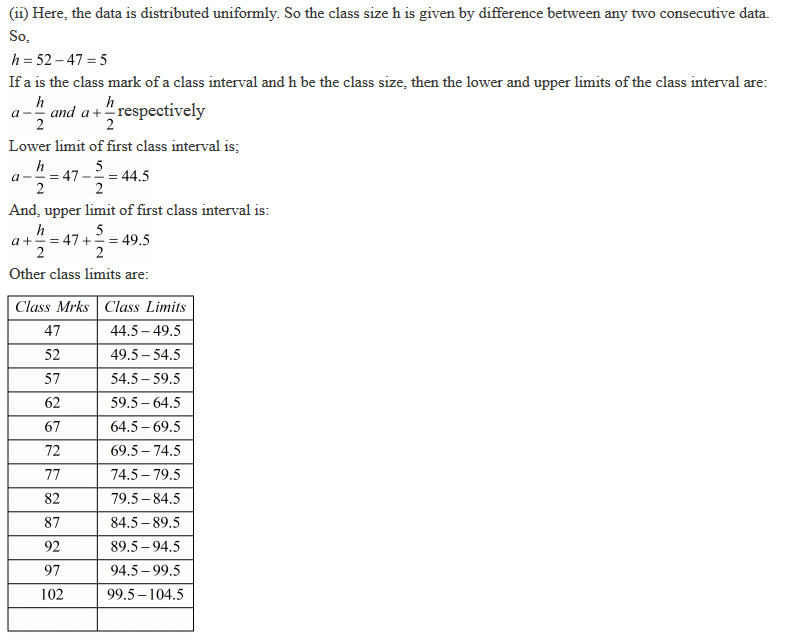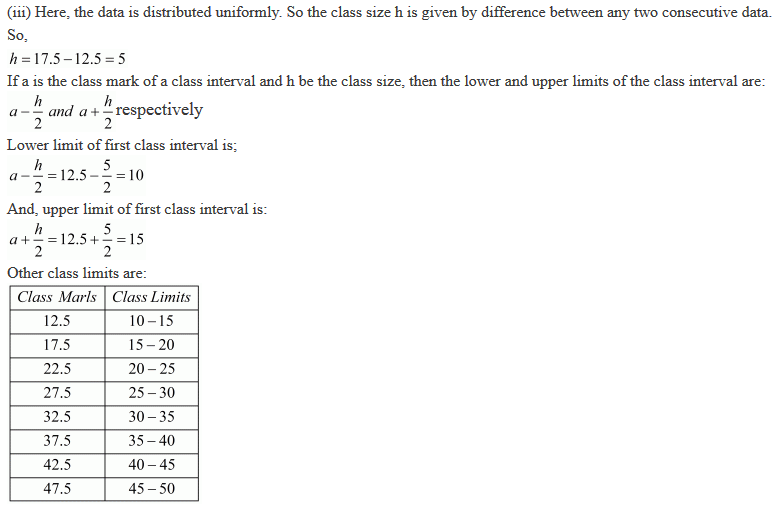14. Following data gives the number of children in 40 families:
1, 2, 6, 5, 1, 5, 1, 3, 2, 6, 2, 3, 4, 2, 0, 0, 4, 4, 3, 2, 2, 0, 0, 1, 2, 2, 4, 3, 2, 1, 0, 5, 1, 2, 4, 3, 4, 1, 6, 2, 2.
Represent it in the form of a frequency distribution.

Solution

Here, the maximum and minimum values of the variate are 6 and 0 respectively.
So the range = 6 - 0 = 6
Here, we will take class size 1. So we must have 6 classes each of size 1.
Therefore, the frequency distribution in which the lower limit is included and upper limit excluded is:15.  The marks scored by 40 students of class IX in mathematics are given below:
81, 55, 68, 79, 85, 43, 29, 68, 54, 73, 47, 35, 72, 64, 95, 44, 50, 77, 64, 35, 79, 52, 45, 54, 70, 83, 62, 64, 72, 92, 84, 76, 63, 43, 54, 38, 73, 68, 52, 54
Prepare a frequency distribution with class size of 10 marks.

Solution

Here, the maximum and minimum values of the variate are 95 and 29 respectively.
So the range = 95 – 29 = 66
Here, we will take class size 10. So we must have 7 classes each of size 10
Lower limit of first class interval is;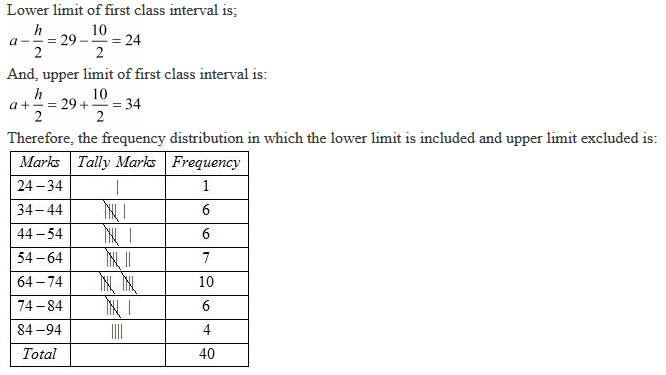16. The heights (in cm) of 30 students of class IX are given below:
155, 158, 154, 158, 160, 148, 149, 150, 153, 159, 161, 148, 157, 153, 157, 162, 159, 151, 154, 156, 152, 156, 160, 152, 147, 155, 163, 155, 157, 153
Prepare a frequency distribution table with 160-164 as one of the class intervals.

Solution

One of the class intervals is 160–164. This means that class size is 4
Here, the maximum and minimum values of the variate are 163 and 147 respectively.
So the range = 163 – 147 = 16
Here, we will take class size 4. So we must have 5 classes each of size 4
Lower limit of first class interval is;17 . The monthly wages of 30 workers in a factory are given below:
83.0, 835, 890, 810, 835, 836, 869, 845, 898, 890, 820, 860, 832, 833, 855, 845, 804, 808, 812, 840, 885, 835, 836, 878, 840, 868, 890, 806, 840, 890.
Represent the data in the form of a frequency distribution with class size 10.

Solution

Here, the maximum and minimum values of the variate are 898 and 804 respectively.
So the range = 898 – 804 = 94
Here, we will take class size 10. So we must have 94/10 i.e. 10 classes each of size 10.
Therefore, the frequency distribution in which the lower limit is included and upper limit excluded is:
Lower limit of first class interval is;18. The daily maximum temperatures (in degree celsius) recorded in a certain city during the month of November are as follows:

25.8, 24.5, 25.6, 20.7, 21.8, 20.5, 20.6, 20.9, 22.3, 22.7, 23.1, 22.8, 22.9, 21.7, 21.3, 20.5, 20.9, 23.1, 22.4, 21.5, 22.7, 22.8, 22.0, 23.9, 24.7, 22.8, 23.8, 24.6, 23.9, 21.1
Represent them as a frequency distribution table with class size 1°C.

Solution

Here, the maximum and minimum values of the variate are 25.8 and 20.5 respectively.
So the range = 25.8 – 20.5 = 5.3
Here, we will take class size 1.
Lower limit of first class interval is;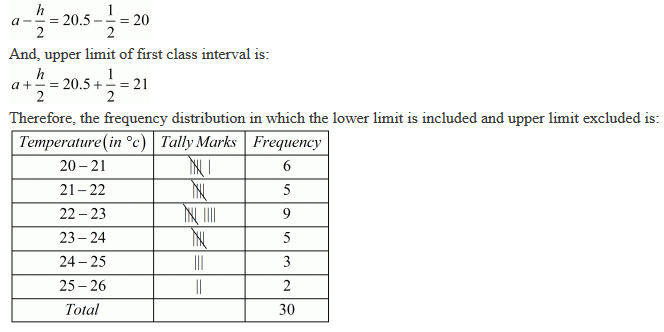19. Construct a frequency table with equal class intervals from the following data on the monthly wages (in rupees) of 28 labourers working in a factory, taking one of the class intervals as 210-230 (230 not included):
220, 268, 258, 242, 210, 268, 272, 242, 311, 290, 300, 320, 319, 304, 302, 318, 306, 292, 254, 278, 210, 240, 280, 316, 306, 215, 256, 236.

Solution

Here, the maximum and minimum values of the variate are 320 and 210 respectively.
So the range = 320 – 210 = 110
Here, we will take class size 20 (As one class interval is 210–230).
Therefore, the frequency distribution in which the lower limit is included and upper limit excluded is:20. The daily minimum temperatures in degrees Celsius recorded in a certain Arctic region are as follows:
− 12.5, −10.8, −18.6, −8.4, −10.8, −4.2, −4.8, −6.7, −13.2, −11.8, −2.3, 1.2, 2.6, 0, −2.4, 0, 3.2, 2.7, 3.4, 0, −2.4, −2.4, 0, 3.2, 2.7, 3.4, 0, −2.4, −5.8, −8.9, −14.6, −12.3, −11.5, −7.8, −2.9.
Represent them as frequency distribution table taking −19.9 to − 15 as the first class interval.

Solution

Since the first class is –19.9 to –15 .
Therefore, the frequency distribution in which the lower limit is included and upper limit excluded is:21.  The blood groups of 30 students of class VIII are recorded as follows:
A, B, O, O, AB, O, A, O, B, A, O, B, A, O, O,
A, AB, O, A, A, O, O, AB, B, A, O, B, A, B, O
Represent this data in the form of a frequency distribution table. Find out which is the most common and which is the rarest blood group among these students

Solution

It can be observed that 9 students have their blood group as A, 6 as B, 3 as AB, and 12 as O.
Therefore, the blood group of 30 students of the class can be represented as follows.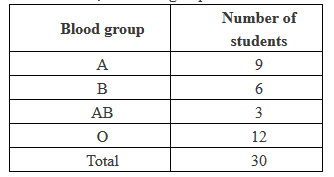It can be observed clearly that the most common blood group and the rarest blood group among these students is O and AB respectively as 12 (maximum number of students) have their blood group as O, and 3 (minimum number of students) have their blood group as AB.

22. Three coins were tossed 30 times. Each time the number of heads occurring was noted down as follow:
0 1 2 2 1 2 3 1 3 0
1 3 1 1 2 2 0 1 2 1
3 0 0 1 1 2 3 2 2 0
Prepare a frequency distribution table for the data given above.

Solution23. Thirty children were asked about the number of hours they watched T.V. programmes in the previous week. The results were found as follows:
1, 6, 2, 3, 5, 12, 5, 8, 4, 8
10, 3, 4, 12, 2, 8, 15, 1, 17, 6
3, 2, 8, 5, 9, 6, 8, 7, 14, 12
(i) Make a grouped frequency distribution table for this data, taking class width 5 and one of the class intervals as 5-10.
(ii) How many children watched television for 15 or more hours  a week?

Solution

(i) Our class intervals will be 0 − 5, 5 − 10, 10 −15…..
The grouped frequency distribution table can be constructed as follows.(ii) The number of children who watched TV for 15 or more hours a week is 2 (i.e., the number of children in class interval 15 − 20).